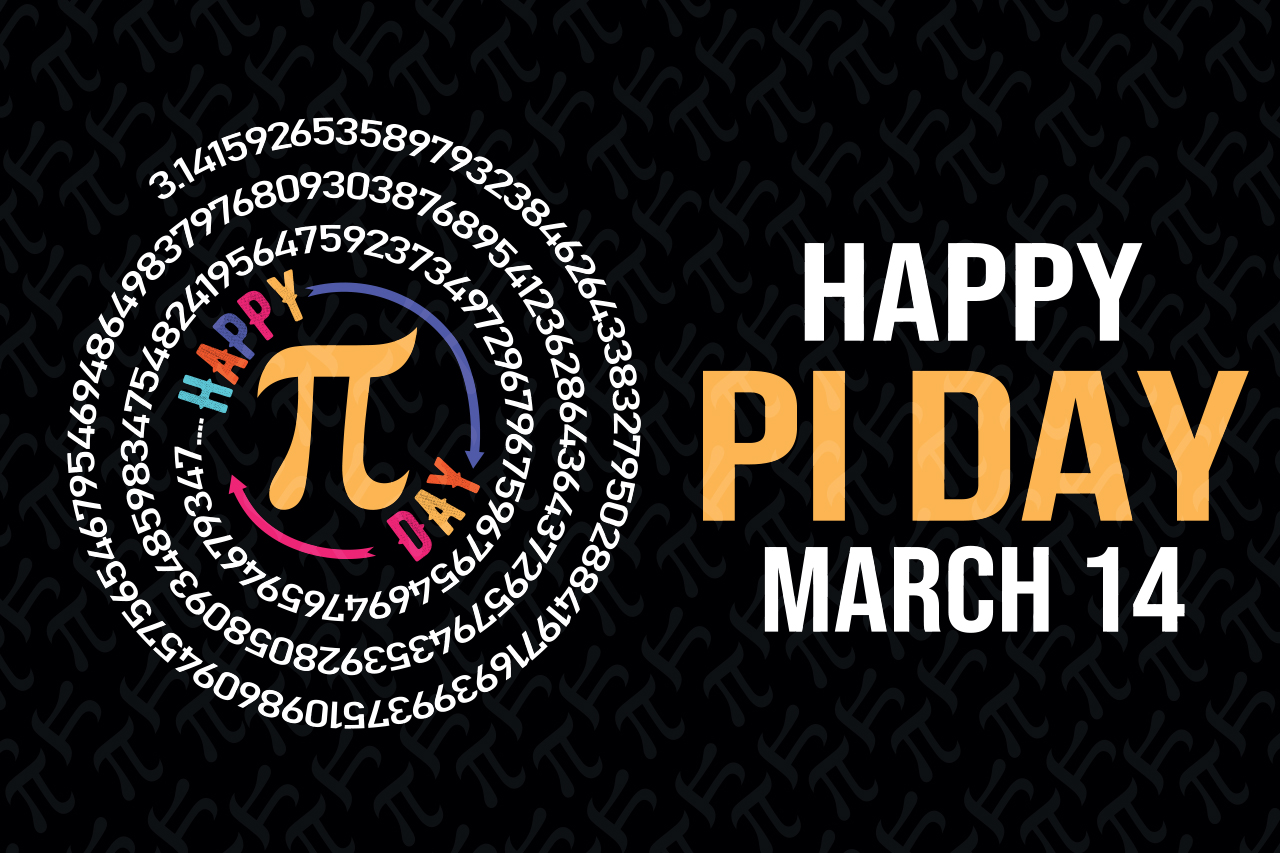Insights

# Happy Pi Day!

## Celebrate Pi Day by exploring the intriguing correlation between pi, the bending of light, and its significance in fiber optic technology. Discover how this mathematical constant plays a vital role in advancing communication and connectivity.

Happy Pi Day! Today, we celebrate the famous number pi (π), roughly 3.14159. You've probably heard of it as the special number that helps us understand circles. But did you know there is a fun connection between how light bends and the number pi?

Pi (π): a mathematical constant that represents the ratio of the circumference of a circle to its diameter

First, let's start with the basics. Pi (π) is a mathematical constant that represents the ratio of the circumference of a circle to its diameter. The value of π is approximately equal to 3.14159, but it is a non-repeating, non-terminating decimal calculated to trillions of digits. Pi is used in a wide range of scientific and technological applications, including fiber optic technology.

To understand the connection between pi and the bending of light, we first need to know a little about how light travels. When light passes through different materials, like from air to water, it bends. This bending is called refraction. The amount that light bends depends on the material and is described by a number called the refraction index.

Refraction Index (also known as the index of refraction): a dimensionless quantity that describes how light propagates through a particular medium. It is defined as the ratio of the speed of light in a vacuum (c) to the speed of light in a medium (v). A higher refraction index indicates that light travels more slowly through the medium, resulting in increased bending or refracting of light rays. n = c/v

Fiber optics work by bouncing light through the strands. The light can only travel through the strand if it enters at a specific angle. This angle depends on the refraction index of the strand's core and the surrounding material, called the cladding layer.

This means, the refractive index of the core is an important factor in determining the speed of data transmission in fiber optics. The higher the refractive index of the core, the slower the speed of light within the core. This is because the higher refractive index causes the light to bend more, which means it has to travel a longer path to reach the other end of the cable.

The refractive index of a material is defined as the ratio of the speed of light in a vacuum to the speed of light in the material. Therefore, the speed of light in the material is inversely proportional to its refractive index. This means that the higher the refractive index, the slower the speed of light.

So how exactly does pi come into play?

The speed of light inside a fiber optic cable can be determined by considering the refractive indices of the core and cladding materials. In this computation, the mathematical constant π plays a role, since the core's refractive index influences both the angle at which light enters and the angle at which it bends while traveling through the core.

But why is this important?

The numerical value of pi has been calculated to trillions of digits, and this precision is crucial for many scientific and technological applications. By calculating the speed of light within the core using pi and the refractive index, engineers can optimize the design of fiber optic cables for faster and more reliable internet access. This ensures that the speed of light within a fiber optic cable remains constant and predictable, which is essential for reliable data transmission.

But let's take a step back from the technicalities and appreciate the wonder of pi. Pi is an irrational number, meaning it cannot be expressed as a finite decimal or a fraction. It goes on forever, never repeating and never ending. Yet, as we saw, its precision is essential for so many applications, including fiber optics. Pi is a universal constant that applies to all circles, making it a fundamental concept in geometry and trigonometry. It is also a mathematical constant that has captured the imagination of people throughout history, from ancient civilizations to modern scientists and mathematicians.

Why should you never talk to π? Because he'll just go on forever.

Pi Day, celebrated annually on March 14th (3/14), is a day to celebrate this amazing mathematical constant and its importance in the world of science and technology. Today, people worldwide come together to celebrate pi with fun activities, pi-themed foods, and of course, plenty of math puns.

The correlation between pi and the refractive index is essential in fiber optic technology, which is vital for faster and more reliable internet access. The precision and universality of pi enable engineers to design and optimize fiber optic cables for the most efficient data transmission. So, as we celebrate Pi Day, let's take a moment to appreciate the wonder of pi and its role in shaping our world.